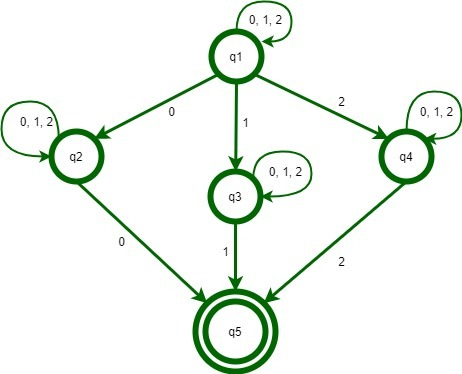# NFA which accepts set of strings over an alphabet {0, 1, 2} such that the final digit has appeared before

Prerequisite – Introduction of Finite Automata
C++ Program to construct an NFA which accepts the set of strings over an alphabet {0, 1, 2} such that the final digit has appeared before.

Examples :

```Input : 01101
Output : Accepted

Input : 012
Output : Not Accepted

Input : 2
Output : Not Accepted

Input : 0122
Output : Accepted ```

Explanation:
In the first example, 01101, the last digit ‘1’ occurred at letter number 2 and 3 of the string. Hence it is accepted. In second example, 012, the occurrence of ‘2’ is only at the last place. Hence it is rejected. Similarly with the third example, 2 is rejected. In the last example, the last digit ‘2’ occured befor end of the string hence it is Accepted.

Approach:

1. Construct a start state.
2. Construct 3 states for input of 0, 1 and 2.
3. Repeat the loops in all state for all the inputs.
4. Connect all the state with a final state.

NFA State Transaction Diagram:Implementation :

 `#include ` `using` `namespace` `std; ` ` `  `// function of state one or starting state ` `void` `q1(string s, ``int` `pos, ``int` `len);  ` ` `  `// function of state two ` `void` `q2(string s, ``int` `pos, ``int` `len); ` ` `  `// function of state three  ` `void` `q3(string s, ``int` `pos, ``int` `len);  ` ` `  `// function of state four ` `void` `q4(string s, ``int` `pos, ``int` `len);  ` ` `  `// function of state five ` `void` `q5(string s, ``int` `pos, ``int` `len);  ` ` `  `// See diagram for help ` ` `  `vector states; ` `int` `accepted = 0; ` ` `  `// Uncomment this function and the function calls to see ` `// the path of string from the start state to end state ` `/* ` `void printVector() ` `{ ` `    ``for (auto i = states.begin(); i != states.end(); i++) ` `        ``cout << *i << " "; ` `    ``cout << endl; ` `} ` `*/` `void` `q5(string s, ``int` `pos, ``int` `len) ` `{ ` `    ``states.push_back(``"Q5->"``); ` `    ``if` `(pos == len) { ` `        ``// printVector(); ` `        ``accepted = 1; ` `    ``} ` `    ``else` `{ ` `        ``states.push_back(``"Dead"``); ` `        ``// printVector(); ` `        ``states.pop_back(); ` `    ``} ` `    ``states.pop_back(); ` `    ``return``; ` `} ` ` `  `void` `q4(string s, ``int` `pos, ``int` `len) ` `{ ` `    ``states.push_back(``"Q4->"``); ` `    ``if` `(pos == len) { ` `        ``// printVector(); ` `        ``states.pop_back(); ` `        ``return``; ` `    ``} ` `    ``if` `(s[pos] == ``'2'``) ` `        ``q5(s, pos + 1, len); ` `    ``q4(s, pos + 1, len); ` `    ``states.pop_back(); ` `    ``return``; ` `} ` ` `  `void` `q3(string s, ``int` `pos, ``int` `len) ` `{ ` `    ``states.push_back(``"Q3->"``); ` `    ``if` `(pos == len) { ` `        ``// printVector(); ` `        ``states.pop_back(); ` `        ``return``; ` `    ``} ` `    ``if` `(s[pos] == ``'1'``) ` `        ``q5(s, pos + 1, len); ` `    ``q3(s, pos + 1, len); ` `    ``states.pop_back(); ` `    ``return``; ` `} ` ` `  `void` `q2(string s, ``int` `pos, ``int` `len) ` `{ ` `    ``states.push_back(``"Q2->"``); ` `    ``if` `(pos == len) { ` `        ``// printVector(); ` `        ``states.pop_back(); ` `        ``return``; ` `    ``} ` `    ``if` `(s[pos] == ``'0'``) ` `        ``q5(s, pos + 1, len); ` `    ``q2(s, pos + 1, len); ` `    ``states.pop_back(); ` `    ``return``; ` `} ` ` `  `void` `q1(string s, ``int` `pos, ``int` `len) ` `{ ` `    ``states.push_back(``"Q1->"``); ` `    ``if` `(pos == len) { ` `        ``// printVector(); ` `        ``states.pop_back(); ` `        ``return``; ` `    ``} ` `    ``if` `(s[pos] == ``'0'``) ` `        ``q2(s, pos + 1, len); ` `    ``else` `if` `(s[pos] == ``'1'``) ` `        ``q3(s, pos + 1, len); ` `    ``else` `if` `(s[pos] == ``'2'``) ` `        ``q4(s, pos + 1, len); ` ` `  `    ``q1(s, pos + 1, len); ` `    ``states.pop_back(); ` `    ``return``; ` `} ` ` `  `int` `main() ` `{ ` `    ``string s; ` `    ``// cin >> s; ` `    ``s = ``"01101"``; ` ` `  `    ``int` `pos = 0; ` `    ``q1(s, pos, s.length()); ` ` `  `    ``if` `(accepted) ` `        ``cout << ``"Accepted"` `<< endl; ` `    ``else` `        ``cout << ``"Not Accepted"` `<< endl; ` `    ``return` `0; ` `} `

Attention reader! Don’t stop learning now. Get hold of all the important CS Theory concepts for SDE interviews with the CS Theory Course at a student-friendly price and become industry ready.

My Personal Notes arrow_drop_upCheck out this Author's contributed articles.

If you like GeeksforGeeks and would like to contribute, you can also write an article using contribute.geeksforgeeks.org or mail your article to contribute@geeksforgeeks.org. See your article appearing on the GeeksforGeeks main page and help other Geeks.

Please Improve this article if you find anything incorrect by clicking on the "Improve Article" button below.

Article Tags :

Be the First to upvote.

Please write to us at contribute@geeksforgeeks.org to report any issue with the above content.